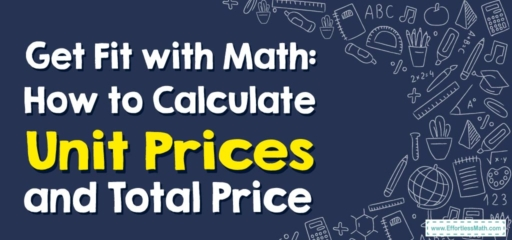# Get Fit with Math: How to Calculate Unit Prices and Total Price

Hello, fitness enthusiasts!

Today, we're not just training our bodies, but also our minds! Whether we're buying protein powder, dumbbells, or new sneakers, understanding unit prices and total price is key to staying financially fit. Lace up your trainers and let's hit the math gym!## 1. Fitness Foundations: Understanding Unit Prices and Total Price

Before we break a sweat, let’s warm up with some key terms:

• Unit Price: This is the cost per unit of a product, such as price per kilogram, per pound, or per item.
• Total Price: This is the final cost of all the units of a product you plan to purchase.

## 2. Gym Guide: How to Calculate Unit Prices and Total Price

Now that we’re warmed up, it’s time for the workout!

### Fitness Coach Guide: Calculating Unit Prices and Total Price

#### Step 1: Identify the Total Cost and the Number of Units

First, find out the total cost of the product and the number of units included.

#### Step 2: Calculate the Unit Price

Next, divide the total cost by the number of units to get the unit price.

#### Step 3: Calculate the Total Price

Finally, to calculate the total price of a desired number of units, multiply the unit price by the number of units you wish to purchase.

For instance, if you’re buying a $$2$$-pound bag of protein powder for $$15$$ and want to know the price per pound and the cost of 5 pounds, what would you do?

1. Identify the Total Cost and the Number of Units: The total cost is $$15$$ for $$2$$ pounds.
2. Calculate the Unit Price: The unit price is $$15$$ divided by $$2$$, or $$7.50$$ per pound.
3. Calculate the Total Price: The total price for $$5$$ pounds is $$7.50$$ times $$5$$, or $$37.50$$.

Great job, fitness friends! Now, not only are you training your body, but you’re also flexing your math muscles. Remember, every rep counts, both in the gym and in your budget! Stay fit, stay frugal, and see you at the next workout!

### What people say about "Get Fit with Math: How to Calculate Unit Prices and Total Price - Effortless Math: We Help Students Learn to LOVE Mathematics"?

No one replied yet.

X
30% OFF

Limited time only!

Save Over 30%

SAVE $5 It was$16.99 now it is \$11.99